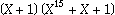These are CRC Guru's answers to the questions you never dared to ask.

 Q: CRC means Cyclic Redundancy Check. But what does that mean? A: The word “cyclic” means that it is based on cyclic codes. “Redundancy” means that something is added to your data which does not increase the amount of information. Q: What is a code in general and a cyclic code in particular? A: A code is a set of words of an alphabet `F` of symbols. A block code is a code where each word has a fixed length `n`. Block codes have the property that there is number `d`, called the minimum distance, such that any two words of the code differ at least in `d` places. This property can be used for error detection and correction: if a code has minimum distance d, then either `d`-1 errors can be detected, or [(`d`-1)/2] errors can be corrected. A cyclic code is a block code for which any shift of a valid code word is also a valid code word. Cyclic codes can be described with polynomials, and in this description they have the property that any code word can be expressed as a multiple of a certain polynomial, called the generator polynomial. The codes we are dealing with here are based on an alphabet of two symbols, ordinary referred to as bits.One thing to remark here is that the data which is processed by a CRC algorithm always has a fixed length, the block length `n`. In practice, this is rarely noticed, because the block length is quite big and leading zeroes are not transmitted. It merely means that there is a maximum message size. Q: Does a CRC polynomial have to be irreducible? A: No. In fact, some popular CRC polynomials are divisible by X+1. Q: Can I choose a CRC polynomial at random? A: No. The polynomial is required to divide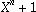, where `n` is the block length of the cyclic code. Q: The Polynomial for CRC-CCITT/XModem is mostly referred to as `0x1021`. But there are also references which say, it is `0x8408`. Which one is correct? A: If interpreted in the right way, both. The CCITT polynomial is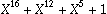, which translates to `0x1021` or to `0x8408`, depending on the bit order chosen. However, it does not make any sense to interpret a bit pattern as a polynomial without specifying the bit order. Therefore, the Catalog of Parameter Sets for Standards which is part of the Painless Guide to CRC Error Detection has certainly a flaw in this point. Q: What is the correct byte order for transmitting the computed CRC? A: Do not attempt to interpret the computed CRC as an integer value. It is a polynomial. So the correct answer is that the byte containing the highest coefficient of the computed polynomial must be transmitted first. When using Ross Williams' reference implementation, this corresponds to the low order byte when “reflection” is used, and to the high order byte when reflection is not used. In CRC++, the result() method automatically delivers the result in a byte order suitable for insertion into the output stream. Q: What is a residual value? A: Some CRC variations invert the result before transmission. In a straightforward implementation, a correct block would then yield a result of L(X), the all-ones-polynomial. However, all CRC algorithms require that the input data stream is multiplied by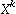, where k is the degree of the CRC polynomial. If the same algorithm is used for verification, then the result for a correct block is not L(X), but the remainder of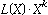. This value is called the residual. It is constant for a given CRC polynomial. In CRC++, the good() method takes care of comparing against the correct value, so you don't have to deal with it.
 To be precise: a polynomial algebra, in which all calculations are done modulo, where `n` is the block length.
 The block size for CRC-CCITT and for CRC-16 is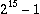bits, but for CRC-32 the block size is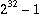bits.
 For example, the CCITT-16/XModem polynomial=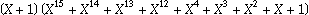The CRC-16 Polynomial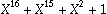=